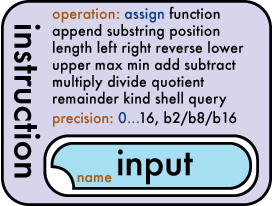### The Instruction Function

The Instruction function is a multi-purpose function that allows interaction and manipulation of inputs and outputs. It can be used for performing arithmetic, string and specialised functionality. Instructions always execute exactly once.

The instruction function is used when you want to perform an operation of some kind on a input or set of inputs.

The most basic instruction operation simply copies data from one store or object into another. Arithmetic operations allow you to perform arithmetic operations such as add and subtract across a set of inputs. The instruction supports a variety of string processing instructions and can also used for some specialised functions such as shell execution and one-time database queries.Instructions must have at least one input. Depending on the operation in use the number of inputs may vary.Operation:
The instruction's operation indicates how input are processed into the outputs. All inputs are operated on left to right (first to last). The following operations are available.

Basic operations:
• assign*
Pushes the input data into the output parameters without changing the data. This is the default value for the instruction operation as denoted by the *asterisk and the blue text in the visual guide.

String processing operations:
• append
Concatenates the data from all the input elements.
• substring
Returns a substring of an initial input value.
• position
Returns the index of the first input in the second input.
• length
Returns the string length of the inputs.
• left
Returns a specified number of characters from the left side of the first input string. Subsequent inputs determine the number of characters.
• right
Returns a specified number of characters from the right side of the first input string. Subsequent inputs determine the number of characters.
• lower
Converts a string into lower case.
• upper
Converts a string into upper case.

Arithmetic operations: (note: the precision of the outputs of these operations can be set using the precision attribute)
Performs an arithmetic add across all the input parameters.
• subtract
Performs an arithmetic subtract across all the input parameters.
• multiply
Performs an arithmetic multiply across all the input parameters.
• divide
Performs an arithmetic divide across all the input parameters and pushes the result into the output parameters. Divide is performed accumulatively first to last.
• quotient
Performs an arithmetic divide across all the input parameters returning the quotient. Arithmetic is performed cumulatively first to last.
• function
Performs a specific task separately from the main program. Also known as a subroutine or subprogram in other languages.
• remainder
Performs an arithmetic modulus (remainder) across all the input parameters. Arithmetic is performed cumulatively first to last.
• max
Returns the maximum value of all input elements.
• min
Returns the minimum value of all input elements.

Special operations:
• shell
Executes a server-side script as specified by the inputs.
• query
Silently executes an SQL statement as specified by the inputs. Returns from the SQL are ignored.
Pecision: (0..16)
All mathematical functions internally occur at the highest precision (16 decimal places). The output is then rounded to the number of decimal places specified. Zero (integer) is the default as denoted by the blue text in the visual guide.

### Basic Instruction Examples

This basic example simply outputs the string "hello world":
``````<instruction>
<input value="hello world" />
</instruction>``````
`hello world`

This is a more verbose version of the above example showing where the default values are incorporated:
``````<instruction operation="assign">
<output space="immediate" />
<input value="hello world" />
</instruction>``````
`hello world`
This examples shows how you would assign a value to a store:
``````<instruction operation="="assign">
<output space="store" value="myVar"/>
<input value="pigeon" />
</instruction>``````
`the store "myVar" contains "pigeon"`
This example shows the instruction function being used to arithmetically add together a number of inputs:
``````<instruction operation="add">
<input value="2" />
<input value="3" />
<input value="4" />
</instruction>``````
`9`
This example shows how the "right" string processing operation is used to trim a string:
``````<instruction operation="right">
<input value="Hippopotamus" />
<input value="8" />
</instruction>``````
`opotamus`

Further examples can be found within the documentation for each operation.